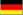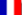## THE ORIGINS OF THE CHRYZODES

In the Platon's works, the circle was already used to represent numbers.
The cathedrals were probably built according to a similar method
A CIRCLE TO DEFINE NUMBERS
For example: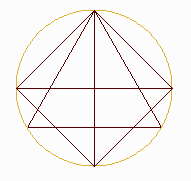To represent number 2 on a circle, a point of origin is determined on the circumference, a second point is placed and  a line joins these points.
To represent number 3, 3 equidistant points are placed and joined and we obtain a triangle. As the zero is the starting point to study the scale of numbers, it's useful to keep the same origin.
To represent number 4, the 4 points are joined and we have a square and a cross.
Above is the obtained figure.
We note that this drawing can also be seen in some middle ages pictures ( Dürer's engravings for example).

Why not repeat the process beyond 4 points ?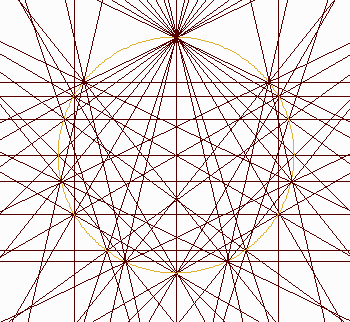Curious as searchers can be, we tried to superpose every star polygon that can be inscribed within a circle whose circumference is divided into 5 points first, then 6 points, 7 points, 8 points, etc.,
In the figure above, every number up to the number 7 are represented.
In the figure below, every number up to the number 12 is represented, that is the superposition of every star polygon that can be inscribed in circles divided into from 2 to 12 points.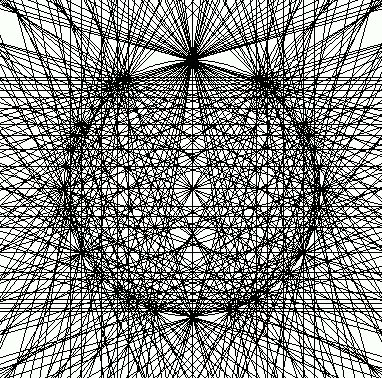Click on the picture